# 22. Mutual Fund Beta, SD, and Sharpe Ratio

## 22.1 – Beta

Over the last few chapters, we discussed various attributes of a mutual fund. We will continue the same in this chapter and focus on key risk measures of a mutual fund. Risk measures include various attributes such as –

• Beta
• Alpha
• Standard Deviation
• Sharpe RatioOne of the key attributes of the mutual fund is the ‘beta’ of the fund. The beta of a mutual fund is the measure of relative risk, expressed as number; Beta can take any value above or below zero. Beta gives us a perspective of the relative risk of the mutual fund vis a vis its benchmark.

I’ll not get into the details of how beta is calculated, I’ve done that in the Future’s module. Here is the link if you are interested to know the math behind –

https://zerodha.com/varsity/chapter/hedging-futures/

Section 11.5 of this chapter discusses the beta in detail. For this chapter, I’ll restrict myself to the application of beta and how you need to use this number. Have a look at the snapshot below –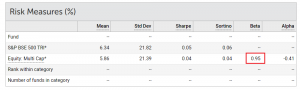I’ve captured this from Value research; these attributes belong to Tata Multicap fund. As you can see, the fund is benchmarked against S&P BSE 500 TRI.

I’ve highlighted the beta of the fund, which is 0.95. Like I mentioned earlier, beta gives us a measure of the relative risk of the fund. In general,

If the beta of a mutual fund is less than 1, then the fund is perceived as less risky compared to its benchmark. For example, the Tata Multicap fund has a beta of 0.95, hence the fund is slightly less risky compared to its benchmark. I say slightly because it’s very close to 1. This implies,  if S&P Sensex 500 falls by  1%, then Tata Multicap fund is expected to fall by 0.95%.

If the beta was 0.6 or 0.65, the fund is less risk or less volatile compared to its benchmark. Why? Because if S&P Sensex 500 falls by  1%, then Tata Multicap fund is expected to fall by only 0.65% and not 0.95%.

This is what I mean by ‘relative risk’; it gives us a perspective of how risky the fund is compared to its benchmark.

Now, if the beta of a mutual fund is equal to 1, then it means the fund is as risky as its benchmark. For example, if the benchmark falls by 1%, the fund is expected to fall by 1%. So both the benchmark and the fund are expected to have similar risk profiles.

Lastly, if the beta of the fund is higher than 1, it implies that the fund is risker compared to its benchmark. For instance, a beta of 1.2 suggests that the fund is 20% riskier compared to its benchmark. If the benchmark falls by 1%, the fund is expected to fall by 1.2%.

When you are looking at the Beta of a stock or an MF, it is very important to recognize the fact that the beta is a measure of relative risk, it tells us how risky the stock or MF is compared to its benchmark. Beta is not an indicator of the inherent risk of the stock or MF.

To put this in context, think about it this way, Ferrari is faster compared to a BMW, this comparison is like the beta. We measure the speed of car one against car two. But does this give you any indication of how fast the Ferrari is? Not really.

Likewise, while beta gives us a perspective of the relative riskiness of an asset, it does not give us the absolute or the inherent risk of the asset itself.

By now, you must have built your perception of beta. Let me ask you this – if a mutual fund has a high beta, do you think it is bad?

Well, the good, bad, ugly part of beta depends on another metric called the ‘Alpha’.

## 22.2 – Alpha

In the previous chapter, we briefly discussed alpha. We defined alpha as the excess return of the fund over and above the benchmark returns. Well, that is true, but we need to make a few small changes to that equation and include our newly introduced friend, beta. To understand alpha, we need to understand the concept of ‘Risk-free’ return. The risk-free return is the maximum return you can generate without taking any risk. By risk I mean – market risk, credit risk, interest rate risk, and unsystematic risk.

There are two return sources which fit in the above definition  – (1) The return from the savings bank account (2) The fixed deposit return.

Of course, we can argue that the banks too are not safe and comes with some degree of risk. Understandable, but let’s keep that argument aside for this discussion 😊

Or if you are a stickler for definitions, let us stick to the treasury bills, issued by the Govt of India. The treasury bills have an implicit sovereign so its deemed safe.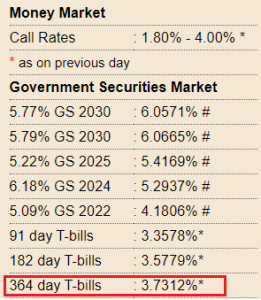The T bill rates as of today are roughly about 3.75%, and let us keep 4% for convenience.

Alpha is defined as the excess return of the mutual fund over the benchmark return, on a risk-adjusted basis.

Risk-adjusted basis means we need to –

• Calculate the difference between the mutual fund returns and the risk-free return
• Calculate the difference between the benchmark return and the risk-free return, multiply this by the beta
• Take the difference between 1 and 2

Mathematically,

Alpha = (MF Return – riskfree return) – (Benchmark return – riskfree return)*Beta

Lets put this in context with an example. Assume a certain fund gives you a return of 10%, its benchmark returns for the same duration is 7%. The beta of the fund is 0.75. What do you think the alpha assuming the risk-free rate is 4%?

Let’s apply the for formula and check –

Alpha = (10%-4%)-(7%-4%)*0.75

= 6% – 2.25%

= 3.75%

As you can see, the alpha is not just the difference between the fund and its benchmark, which if true, the alpha would have been –

10% – 7%

=3%

But rather, the alpha is 3.75%.

Now, many of you may not find this intuitive. You may question where the additional 0.75% comes from.

Well, think about it, the fund has managed to generate a 10% return compared to the Index’s 7% while managing to stay significantly less volatile (remember beta is is just 0.75). Hence we are rewarding the fund for its good behaviour or less volatile behaviour. Therefore the alpha is 3.75% as opposed to just 3%.

Now, imagine the same fund, with the same returns, but the beta is 1.3 instead of 0.75. What do you think is the alpha?

By now, you should guess that since the beta is high, the fund gets penalised for its erratic behaviour. Therefore the alpha should be lower.

Let us see if the numbers agree to this thought.

Alpha = (10%-4%)-(7%-4%)*1.3

= 6% – 3.9%

= 2.1%

See that? While the returns remain the same, thanks to beta, the alpha is significantly lesser on a risk-adjusted basis.

To conclude, alpha is the excess return of the fund over above the benchmark returns. Alpha is a risk-adjusted. The fund is rewarded if the returns are generated by keeping a low-risk profile and penalized for being volatile.

By now, you must have realized that volatility plays an important role in measuring mutual funds performance. Beta is a measure of volatility; it tells us how risky the fund is when compared to its benchmark. Beta is a relative risk and does not reveal the fund’s inherent risk.

The inherent risk of a fund is revealed by the ‘Standard Deviation’ of the fund.

22.3 – Standard Deviation (SD)

I’ve explained the concept of ‘Standard Deviation’ in details here – https://zerodha.com/varsity/chapter/understanding-volatility-part-1/

I’d suggest you go through that entire chapter to understand the concept of standard deviation and volatility. This will help you not just in your MF investments, but also investments in stocks.

I’ll take the liberty of skipping the explanation of standard Deviation since its already explained. However, if you are in no mood to read an entire chapter to figure out the standard Deviation, then here is your shortcut –

• The standard deviation of a stock or a mutual fund represents the riskiness of the stock or the mutual fund
• Standard Deviation is a percentage, expressed as an annualised figure
• Higher the standard Deviation, higher is the volatility of the asset. Higher the volatility, higher is the risk.

For example, consider these two funds –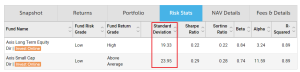I’ve taken the snapshot from Value research. The funds under consideration are the Axis Small-cap fund and Axis long term equity.

The SD of the small-cap fund is 23.95% while the long term equity is 19.33%, which implies that the small-cap fund is way riskier compared to the long term equity fund.

To put this context, if you invest Rs.10,000/- across funds at the same time, then by the end of the year the profit or loss can be anywhere in this range –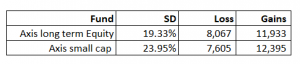Loss = Investment * (1-SD)

Gains = Investment * (1+SD)

The larger the SD, the larger the possibility of loss or gains.

Generally speaking, the SD for mid and small-cap funds are higher compared to large-cap stocks.

Do note, volatility or Standard Deviation should not worry you. Markets are volatile, and equities are volatile, mutual funds are volatile; this is the very nature of markets. So if you can’t fathom watching your investment see-saw between gains and loss, then perhaps you should reconsider your investment decision in equities.

But if you do invest in equities, then you need to learn to manage volatility. There are two ways to deal with this beast called ‘Volatility’ –

• Diversify smartly (and not over diversify)

I think that time is the ultimate antidote against volatility. Give your investments time, and time will take care of volatility. All along with this module, I’ve stressed the importance of giving your MF investments time, and this is the reason why I’ve stressed on it.

Anyway, while at it, check the Alpha and Beta of both these funds. Few observations –

• The beta of both the funds is sub 1, which means compared to their benchmark they are relatively less risky. But how risky are they individually? We can answer this by looking at the SD
• Alpha is a positive number for both the funds, which is a good thing. The Alpha for Axis small-cap fund is quite impressive. I’d guess this is because of the low beta factor plus the low risk-free return prevailing in the economy.

I hope the risk parameters are starting to make sense to you. We will now shift focus to another parameter called the ‘Sharpe Ratio’.

22.4 – Sharpe Ratio

Sharpe Ratio is one of the most sacred formulas in Finance. It was invented by Willam F Sharpe, an American Economist in the year in 1966. He was awarded the Nobel prize in 1990 for his work the Capital asset pricing model.

Assume, there are two large-cap funds -Fund A and Fund B. Here is how they have performed in terms of returns –

Fund A – 14%

Fund B – 16%

Which of the two funds are better? Well, Fund B has a higher return, so without a doubt, Fund B is a better fund.

Now, consider the following –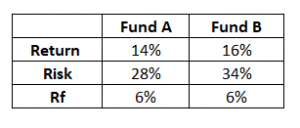Rf is the risk-free return. Along with the fund’s return, I’ve also stated the standard deviation/volatility/risk of the two funds. Now, which of the two funds do you think is better?

I guess it gets a little complex to figure out which these two funds are better given that we have to evaluate them on two parameters, i.e. both the risk and return.

Ignoring the risk, purely on a return basis, Fund B is better. Ignoring the return, purely on a risk basis, Fund A is better. But in reality, you cannot isolate risk and reward; you need to factor in both these and figure out which of these two are better.

The Sharpe Ratio helps us here. It bundles the concept of risk, reward, and the risk-free rate and gives us a perspective.

Sharpe ratio = [Fund Return – Risk-Free Return]/Standard Deviation of the fund

Lets apply the math for Fund A –

= [14% – 6%] / 28%

= 8%/28%

= 0.29

The number tells us that the fund generates 0.29 units of return (over and above the risk-free return) for every unit of risk undertaken. Naturally, by this measure, the higher the Sharpe ratio, the better it is as we all want higher returns for every unit of risk undertaken.

Lets see how this turns out for Fund B –

= [16% – 6% ] / 34%

= 10% / 34%

= 0.29

So it turns out that both the funds are similar in terms of their risk and reward perspective. And there is no advantage of choosing Fund A over Fund B.

Now, instead of 34% standard deviation, assume Fund B’s standard Deviation is 18%.

[16% – 6% ] / 18%

= 10% / 18%

= 0.56

In this case, Fund B is a better choice because Fund B generates more return for every unit of risk undertaken.

Do note, Sharpe ratio considers only price based risk. It does not consider credit or interest rate risk. Hence, there is no point looking at the Sharpe ratio for debt funds.

In the next chapter, I’ll discuss the Sortino’s ratio and the Capture ratios and conclude our discussion on Mutual Fund risk parameters and then shift focus on building Mutual Fund portfolios.

And I promise I’ll put up the next chapter quickly 😊

### Key takeaways from this chapter

• Beta measures the relative risk of the fund compared to its benchmark
• Higher the beta, higher is the relative risk
• Beta is not an indicator of the inherent risk of the fund
• Alpha is the excess return over and above the benchmark return on a risk-adjusted basis
• Higher the beta, lower is the alpha and vice versa
• The standard Deviation measures the riskiness of the fund. Higher the SD, higher is the volatility of the fund
• Sharpe ratio measures the unit of return earned for every unit of risk undertaken
• Higher the Sharpe ratio, better is the fund.

1.Ray says:

Excellent explanation. You made it so easy to understand. Thanks.

•Karthik Rangappa says:

Happy learning 🙂

2.Dr Piyush Vatsa says:

When you start doing things in a much simpler way, after (not before 😉) learning the complex things- you know you are Genius- that defines you Mr Karthik R

•Karthik Rangappa says:

Hahah, thanks for the kind words. That will inspire me to write better 🙂

3.Jatin says:

If beta is a number less than zero (negative number), how do we interpret that wrt benchmark?

•Karthik Rangappa says:

It means that if the benchmark gives a positive return, the fund will give a -ve return. It is very unlikely to find an MF which fits into this category.

4.Mandira says:

Sir, you r excellent and you made investing in simple words. God bless u sir.

•Karthik Rangappa says:

5.S . K . Choudhury says:
•Karthik Rangappa says: